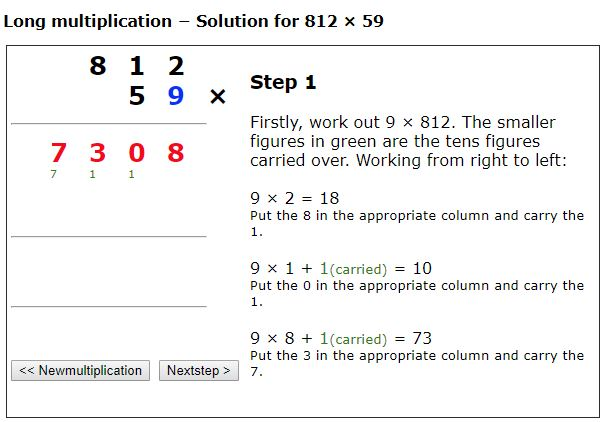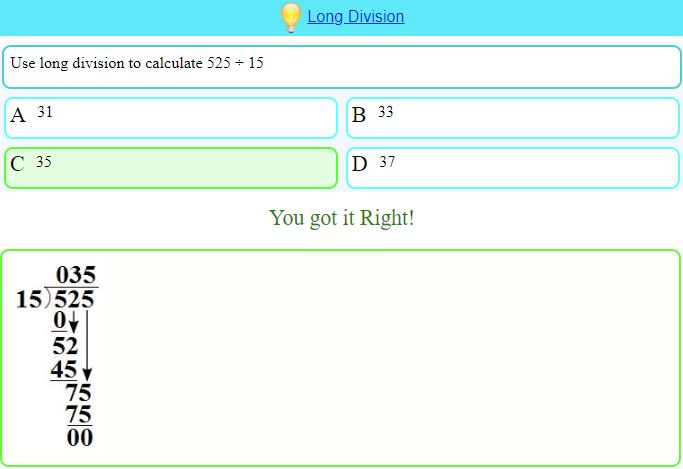# Multiplication and Division

A look at a selection of resources for multiplication and division – from 2×1 to multiplication and division of polynomials!

The tutorial video is very clear on how this Mathigon resource can be used with spaced repetition, there is also, very usefully a Shuffle mode for simple practice.

Choose the Settings and Instructions icon for a summary of the resource and access shuffle mode to practice with a random subset from all decks at once. You can also jump to a later deck with more difficult questions and different visualisations.

For more practice try Transum for several resources for mastering tables, including, for Mixed Practice, Beat the Clock. Level 6 offers mixed multiplication and Division problems, later levels use all 4 operations.

Also from Transum, try Expedite:

For a challenge, try this Open Middle problem from Steve Phelps, Multiplying Two-Digit Numbers.

For a very clear demonstration of the area model for multiplication, see PhET Sims Area Model – Multiplication. You can enter your own calculations and as always with the PhET Simulations choose what you want displayed.

Following on from the area model, for your older students, try Divide it up from Underground Maths, a resource designed to help students to make links between multiplication and division of polynomials using multiplication grids. The problem is presented in the image here, but also provided is a warm-up activity and further notes

The UK National Curriculum specifies that:
Pupils should be taught to:

Year 6 (UK KS2 age 10-11)

• multiply multi-digit numbers up to 4 digits by a two-digit whole number using the formal written method of long multiplication
• divide numbers up to 4 digits by a two-digit whole number using the formal written method of long division, and interpret remainders as whole number remainders, fractions, or by rounding, as appropriate for the context
• divide numbers up to 4 digits by a two-digit number using the formal written method of short division where appropriate, interpreting remainders according to the context

Appendix 1 of the KS2 document includes the examples below and states that “the examples of formal written methods for all four operations illustrate the range of methods that could be taught. It is not intended to be an exhaustive list, nor is it intended to show progression in formal written methods. For example, the exact position of intermediate calculations (superscript and subscript digits) will vary depending on the method and format used. For multiplication, some pupils may include an addition symbol when adding partial products. For division, some pupils may include a subtraction symbol when subtracting multiples of the divisor.

And at KS3 (UK age 11-14) we are reminded that students should be able to “use the four operations, including formal written methods, applied to integers, decimals, proper and improper fractions, and mixed numbers, all both positive and negative”.